# The Fundamental Theorem of Arithmetic

The Fundamental Theorem of Arithmetic

## Table of Contents

 1 Fundamental Theorem of Arithmetic: Definition 2 Fundamental Theorem of Arithmetic: Proof 3 HCF and LCM Using Fundamental Theorem of Arithmetic 4 Thinking out of the Box! 5 Solved Examples 6 Important Notes 7 Practice Questions 8 Maths Olympiad Sample Papers 9 Frequently Asked Questions (FAQs)

We at Cuemath believe that Math is a life skill. Our Math Experts focus on the “Why” behind the “What.” Students can explore from a huge range of interactive worksheets, visuals, simulations, practice tests, and more to understand a concept in depth.

Book a FREE trial class today! and experience Cuemath's LIVE Online Class with your child.

## Fundamental Theorem of Arithmetic: Definition

The statement of Fundamental Theorem Of Arithmetic is:

"Every composite number can be factorized as a product of primes, and this factorization is unique, apart from the order in which the prime factors occur."

For example, let us find the prime factorization of $$240$$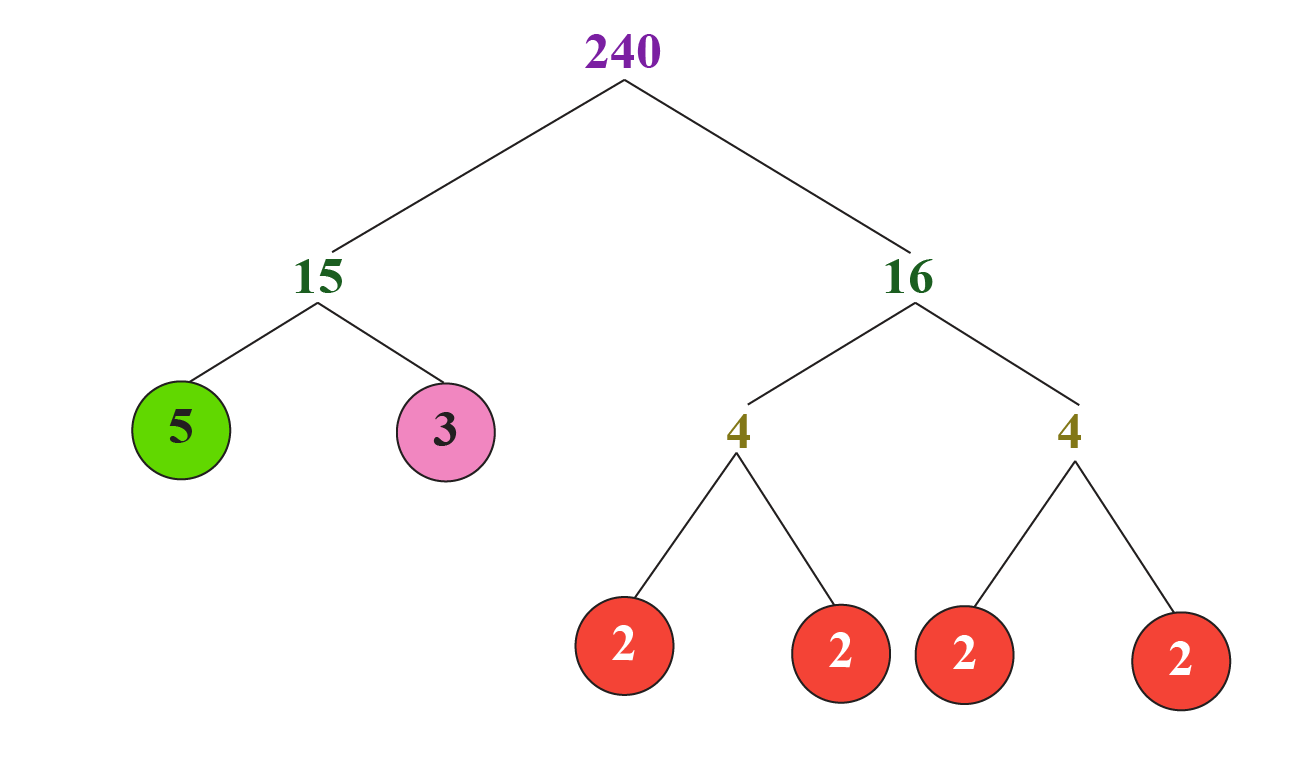From the above figure,\begin{aligned} 240 &=2 \times 2 \times 2 \times 2 \times 3 \times 5 \\ &=2^{4} \times 3^{1} \times 5^{1} \end{aligned}

Our theorem further tells us that this factorization must be unique.

That is, there is no other way to express $$240$$ as a product of primes.

Of course, we can change the order in which the prime factors occur.

For example, the prime factorization can be written as: \begin{aligned} 240 &=3^{1} \times 2^{4} \times 5^{1} \\ &=3^{1} \times 2^{2} \times 5^{1} \times 2^{2} \text { etc. } \end{aligned}

But the set of prime factors (and the number of times each factor occurs) is unique.

That is, $$240$$ can have only one possible prime factorization, with four factors of $$2$$, one factor of $$3$$, and one factor of $$5$$

## Fundamental Theorem of Arithmetic: Proof

To prove the fundamental theorem of arithmetic, we have to prove the existence and the uniqueness of the prime factorization.

Thus, the fundamental theorem of arithmetic: proof is done in TWO steps.

We will prove that for every integer, $$n \geq 2$$, it can be expressed as the product of primes in a unique way:

$n =p_{1} p_{2} \cdots p_{i}$

### Step 1 - Existence of Prime Factorization

We will prove this using Mathematical Induction.

Basic Step: The statement is true for $$n=2$$

Assumption Step: Let us assume that the statement is true for $$n=k$$

Then, $$k$$ can be written as the product of primes.

Induction Step: Let us prove that the statement is true for $$n=k+1$$

If $$k+1$$ is prime, then the case is obvious.

If $$k+1$$ is NOT prime, then it definitely has some prime factor, say $$p$$

Then $k+1=p j, \text{where } j < k \rightarrow (1)$

Since $$j<k$$, by the "inductive step", $$k$$ can be written as the product of primes.

Thus, from (1), $$k+1$$ can also be written as the product of primes.

Thus, by the mathematical induction, the "existence of factorization" is proved.

### Step 2 - Uniqueness of Prime Factorization

Let us assume that $$n$$ can be written as the product of primes in two different ways, say,

\begin{aligned} n &=p_{1} p_{2} \cdots p_{i} \\ &=q_{1} q_{2} \cdots q_{j} \end{aligned}

Since these are prime factorizations, $$q_{1}, q_{2}, \ldots, q_{j}$$ are coprime numbers (as they are prime numbers).

Therefore, by Euclid's Lemma, $$p_1$$ divides only one of the primes.

Note that $$q_1$$ is the smallest prime and so $$p_1=q_1$$

In the same way, we can prove that $$p_n=q_n$$, for all $$n$$

Hence, $$i=j$$

Thus, the prime factorization of $$n$$ is unique.

We can find the prime factorization of any number using the following simulation.

CLUEless in Math? Check out how CUEMATH Teachers will explain The Fundamental Theorem of Arithmetic to your kid using interactive simulations & worksheets so they never have to memorise anything in Math again!

Explore Cuemath Live, Interactive & Personalised Online Classes to make your kid a Math Expert. Book a FREE trial class today!

## HCF and LCM Using Fundamental Theorem of Arithmetic

To find the HCF and LCM of two numbers, we use the fundamental theorem of arithmetic.

For this, we first find the prime factorization of both the numbers. Next, we consider the following:

• HCF is the product of the smallest power of each common prime factor.
• LCM is the product of the greatest power of each common prime factor.

Example:

What is the HCF of $$850$$ and $$680$$?

Solution:

We first find the prime factorizations of these numbers.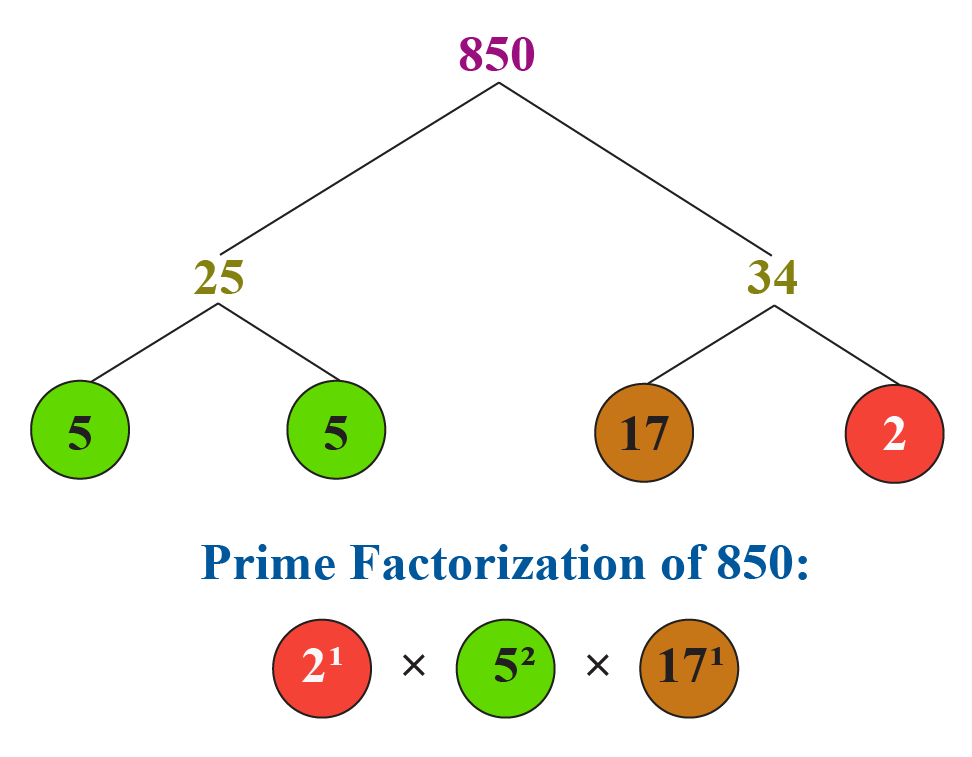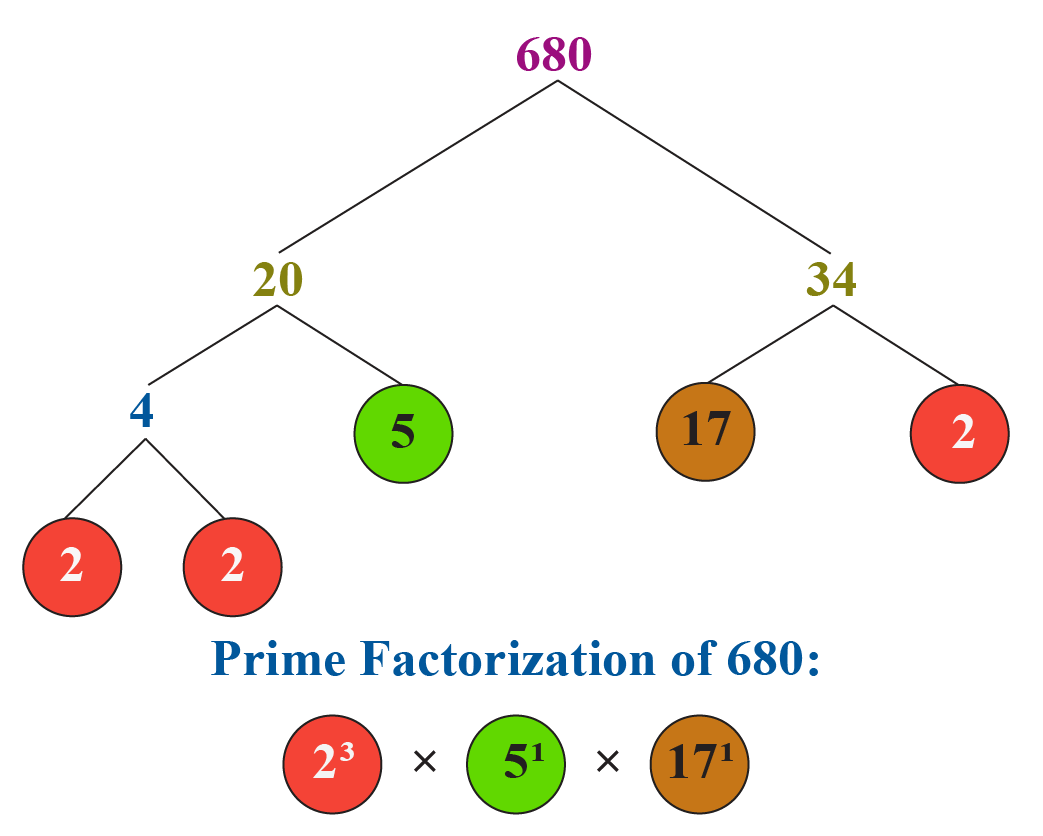Thus:

\begin{align} 850&=2^{1} \times 5^{2} \times 17^{1}\\[0.3cm]680&=2^{3} \times 5^{1} \times 17^{1} \end{align}

HCF is the product of the smallest power of each common prime factor.

Hence,

$\text{HCF }(850, 680) = 2^1 \times 5^1 \times 17^1 = 170$

LCM is the product of the greatest power of each common prime factor.

Hence,

$\text{LCM }(850, 680) = 2^3 \times 5^2 \times 17^1 = 3400$

Thus,

 $\text{HCF }(850, 680) = 170\\[0.3cm]\text{LCM }(850, 680) = 3400$Think Tank

Two positive numbers, $$a$$ and $$b$$ are written as $$a=x^5y^4$$  & $$b=x^2y^3$$; $$x$$ and $$y$$ are prime numbers.

1. Find the HCF $$(a,b)$$
2. Find the LCM $$(a,b)$$

Help your child score higher with Cuemath’s proprietary FREE Diagnostic Test. Get access to detailed reports, customized learning plans, and a FREE counseling session. Attempt the test now.

## Solved Examples

 Example 1

Express $$1080$$ as the product of prime factors. Is this factorization unique?

Solution:

We will find the prime factorization of $$1080$$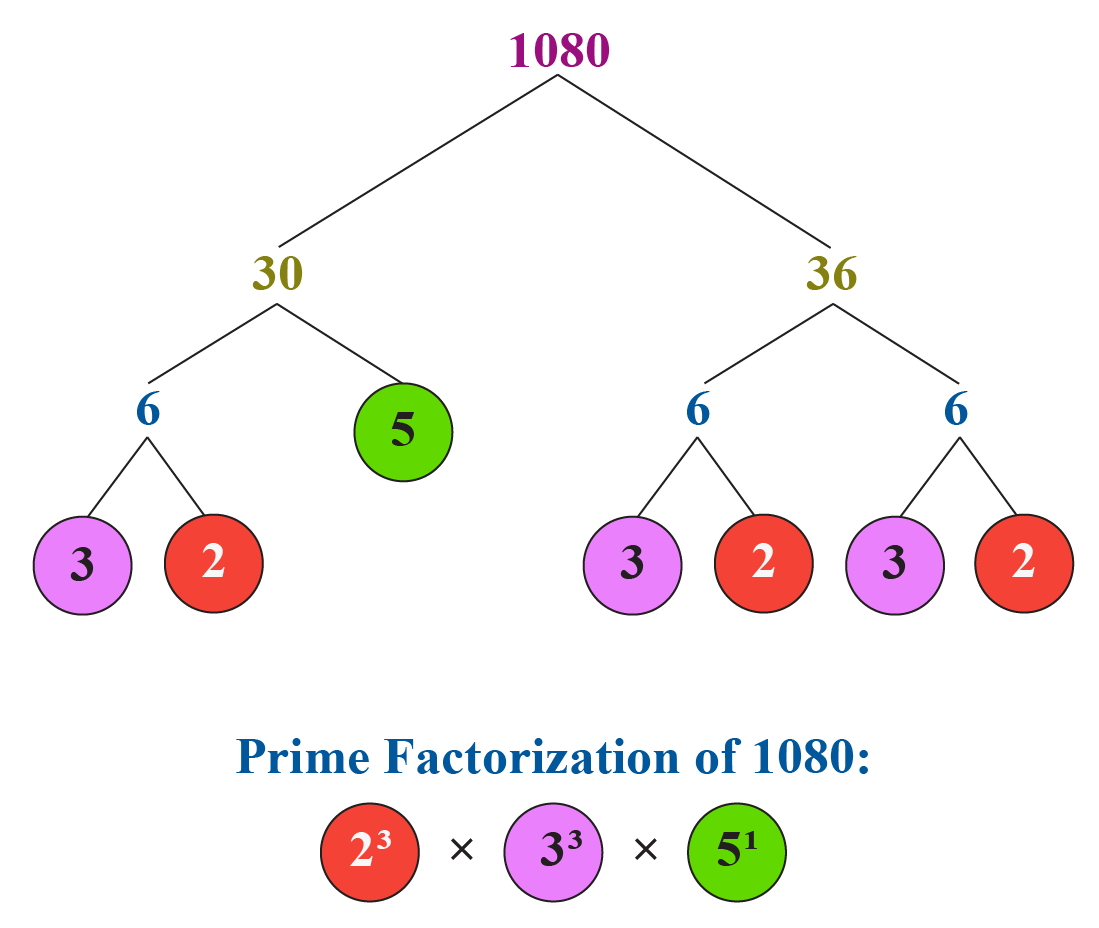Thus,

 $$1080=2^{3} \times 3^{3} \times 5^{1}$$

The above prime factorization is unique by the fundamental theorem of arithmetic.

 Example 2

Find the HCF of $$126, 162$$ and $$180$$ using the fundamental theorem of arithmetic.

Solution:

We will find the prime factorizations of $$126, 162$$ and $$180$$.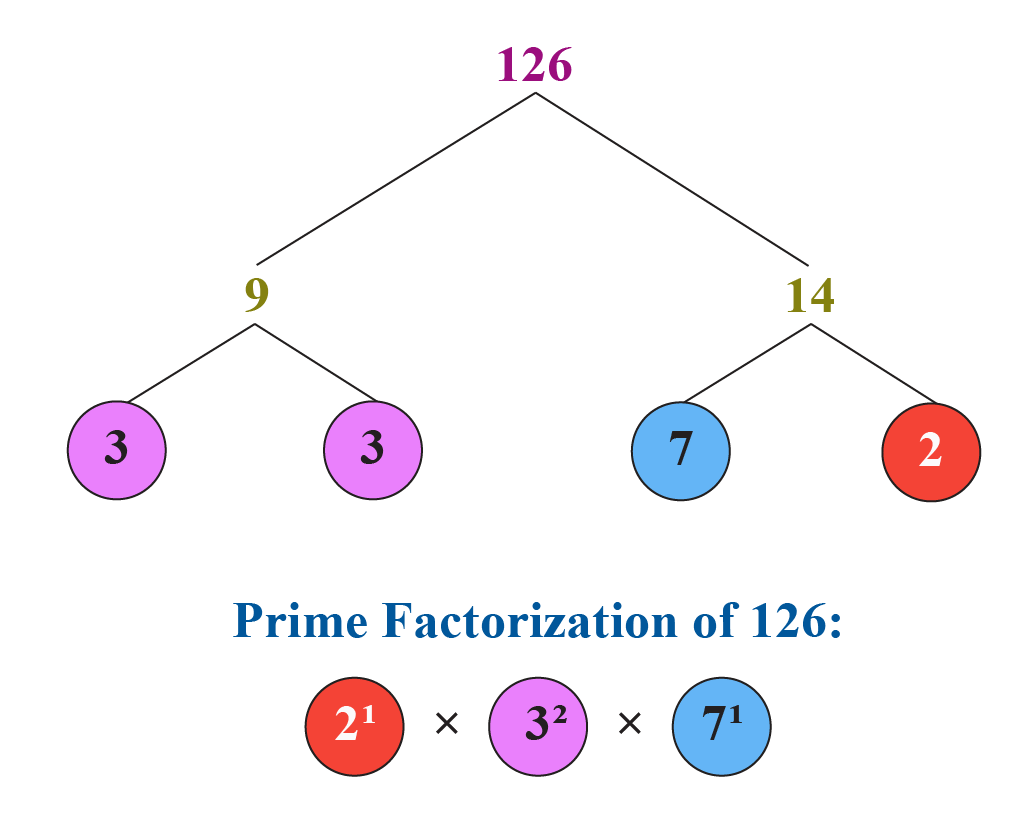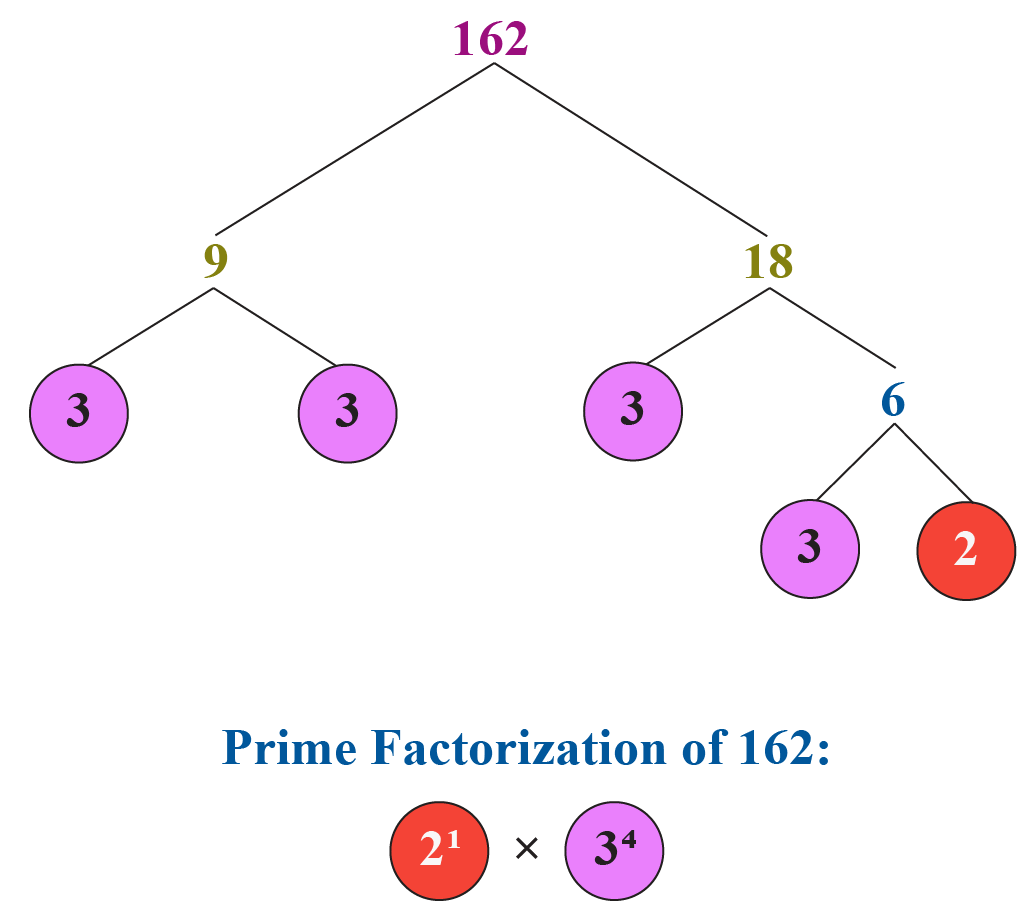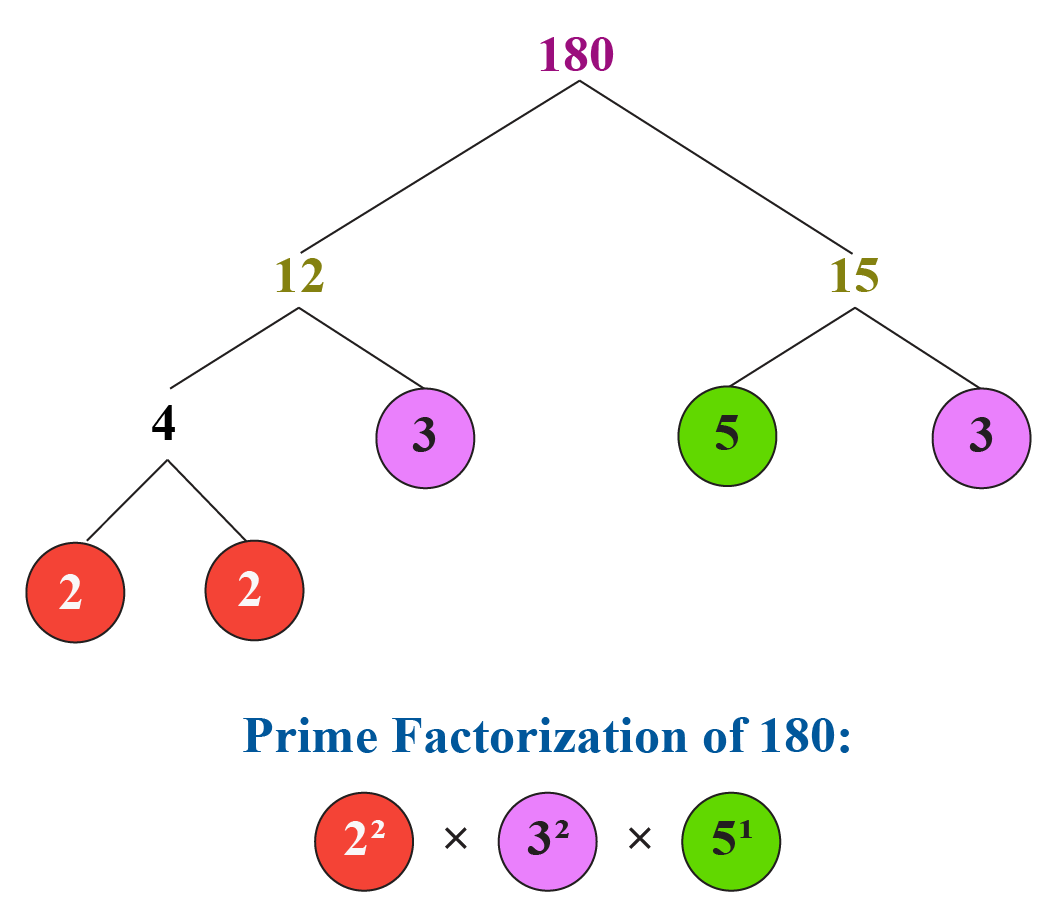Thus,

\begin{align}126&=2^{1} \times 3^{2} \times 7^{1}\\[0.3cm]162&=2^{1} \times 3^{4}\\[0.3cm]180&=2^{2} \times 3^{2} \times 5^{1}\end{align}

The HCF is the product of the smallest power of each common prime factor.

Hence,

$\text{HCF }(126,162,180) = 2^1 \times 3^2 = 18$

 $\text{HCF }(126,162,180) = 18$
 Example 3

Find the LCM of $$48$$ and $$72$$ using the fundamental theorem of arithmetic.

Solution:

We will find the prime factorizations of $$48$$ and $$72$$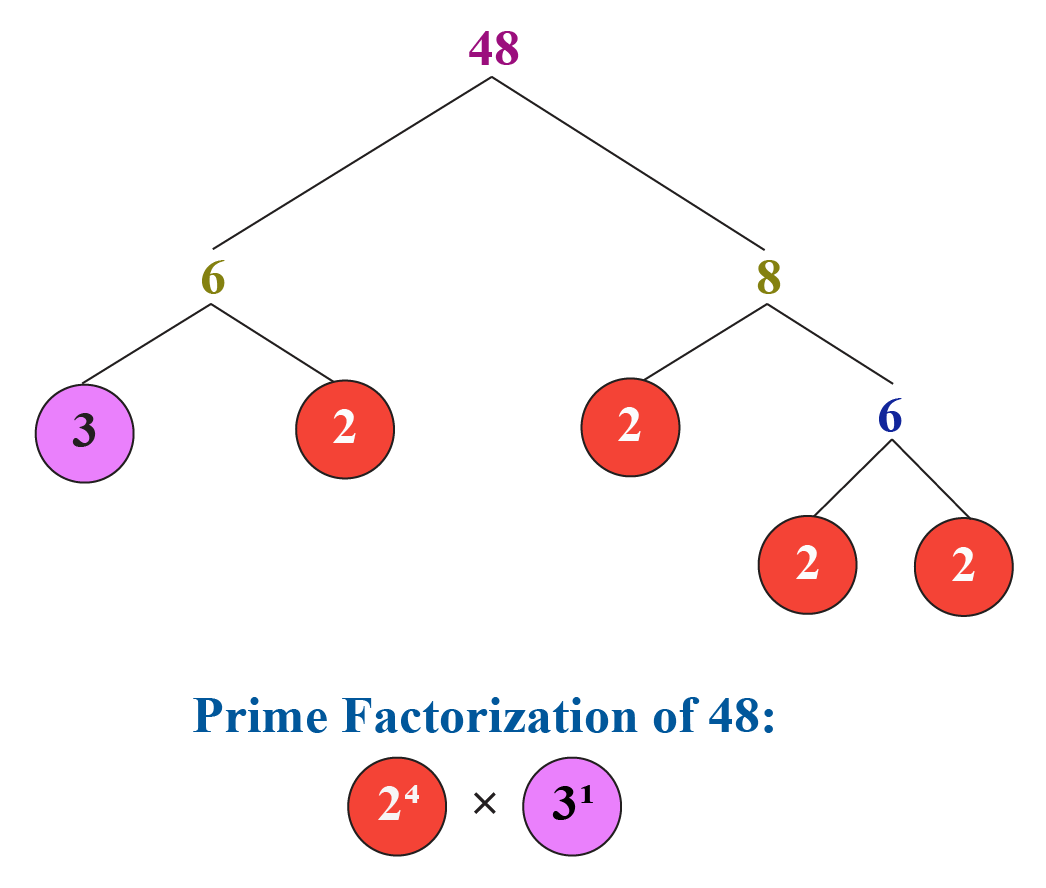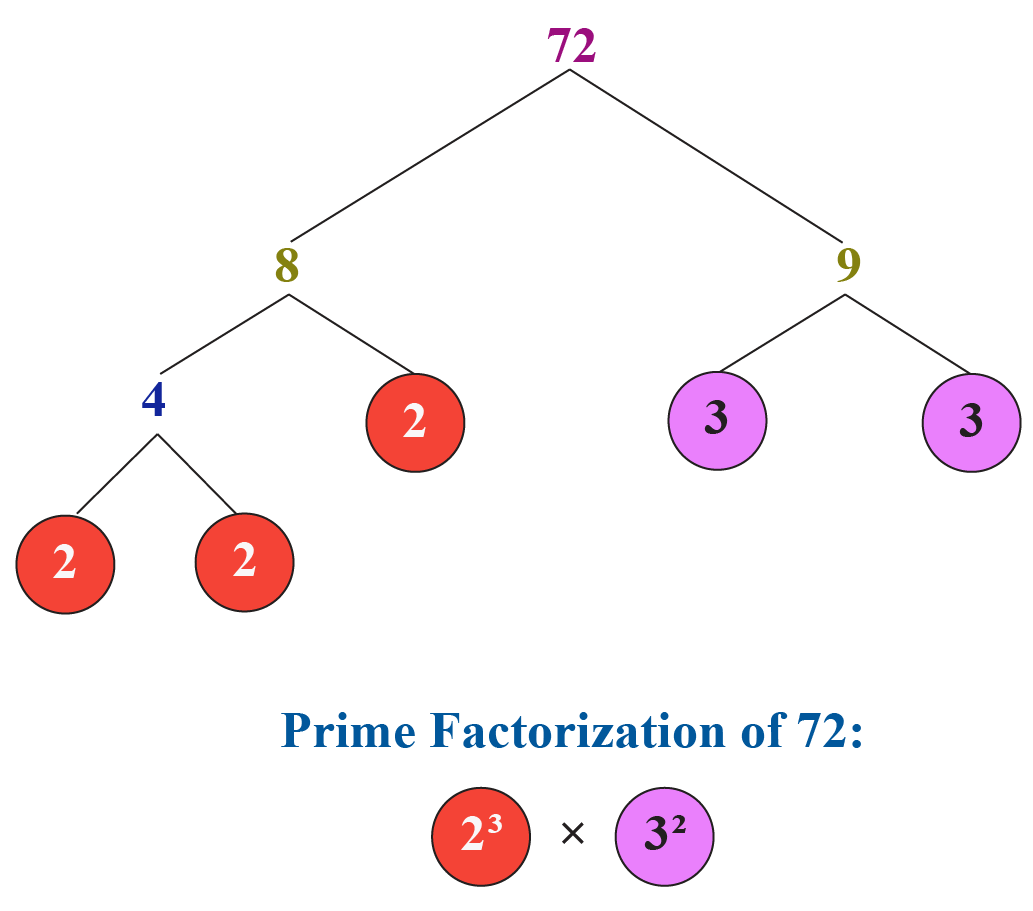The LCM is the product of the greatest power of each common prime factor.

Hence,

$\text{LCM }(48, 72) = 2^4 \times 3^2 = 144$

Thus,

 $\text{LCM }(48, 72) = 144$
Download Arithmetic Integers Worksheets
Arithmetic Integers
grade 10 | Questions Set 2
Arithmetic Integers
grade 10 | Questions Set 1
Arithmetic Integers
grade 10 | Answers Set 1
Arithmetic Integers
grade 10 | Answers Set 2
More Important Topics
Numbers
Algebra
Geometry
Measurement
Money
Data
Trigonometry
Calculus